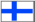Suomeksi

### Nonlinear factor analysis

The models typically used in unsupervised learning assume that the observations have been generated by simple factors, which cannot be directly measured but which influence the observations more or less directly.

According to the model used in the traditional linear factor analysis, the observations are generated by factors, which have Gaussian distributions. The factors are assumed to generate the observations through linear combinations. In addition, the observations are assumed to have Gaussian noise. In many cases, the linearity and gaussianity assumptions are not good at describing the observations, but due to the ease of use, linear factor analysis is widespread particularly in many humanistic sciences.

Unlike in factor analysis, in independent component analysis (ICA) the factors are not assumed to have Gaussian distributions. Computational efficient methods, which can be used instead of the traditional factor analysis, have been developed in the ICA research group in the laboratory of computer and information science.

Another important extension to the ordinary factor analysis is the relaxation of the linearity assumption. In nonlinear factor analysis, the observations are assumed to be generated from the factors through a nonlinear mapping.

Last updated on 15th June, 1999.
<Harri.Lappalainen@hut.fi>